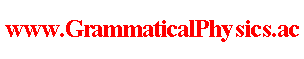since 2006 Help　Sitemap < Forum > < Problems > < Quantum History Theory > < Uda Equation of Dirac Particle > In this page, I investigate a quantum mechanics of a Dirac particle represented by a wave function obeying the Dirac equation: [iγμ∂μ - eγμAμ(x) - m]ψ(x) = 0. iγ0∂0ψ(x) = [-iγk∂k + eγμAμ(x) + m]ψ(x) i∂0ψ(x) = γ0[-iγk∂k + eγμAμ(x) + m]ψ(x) This equation has the same form as the form of Schrodinger equation of three dimensional quantum mechanics with spin. So I can easily make Uda equation of the Dirac equation by the same manner as the manner used when I made the first Uda equation. Author Yuichi Uda, Write start at 2019/04/24/15:45JST, Last edit at 2019/04/24/16:26JST